Скачать презентацию Stat 35 b Introduction to Probability with Applications

907553c17faf7d102592403a80077802.ppt

• Количество слайдов: 8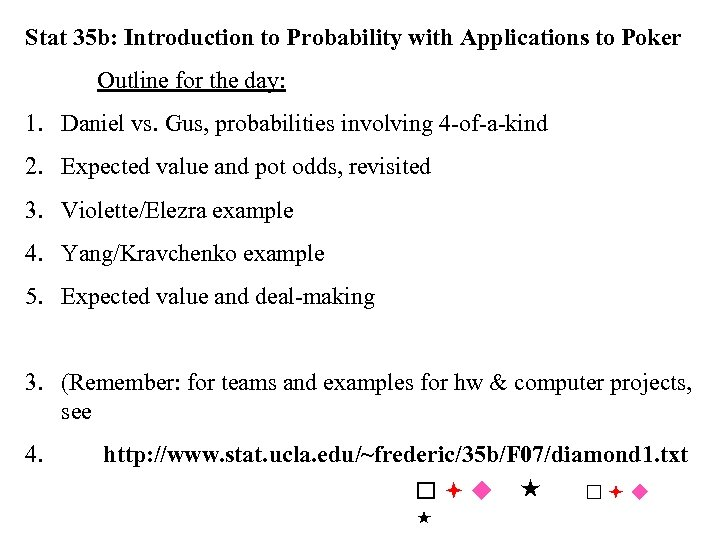Stat 35 b: Introduction to Probability with Applications to Poker Outline for the day: 1. Daniel vs. Gus, probabilities involving 4 -of-a-kind 2. Expected value and pot odds, revisited 3. Violette/Elezra example 4. Yang/Kravchenko example 5. Expected value and deal-making 3. (Remember: for teams and examples for hw & computer projects, see 4. http: //www. stat. ucla. edu/~frederic/35 b/F 07/diamond 1. txt u u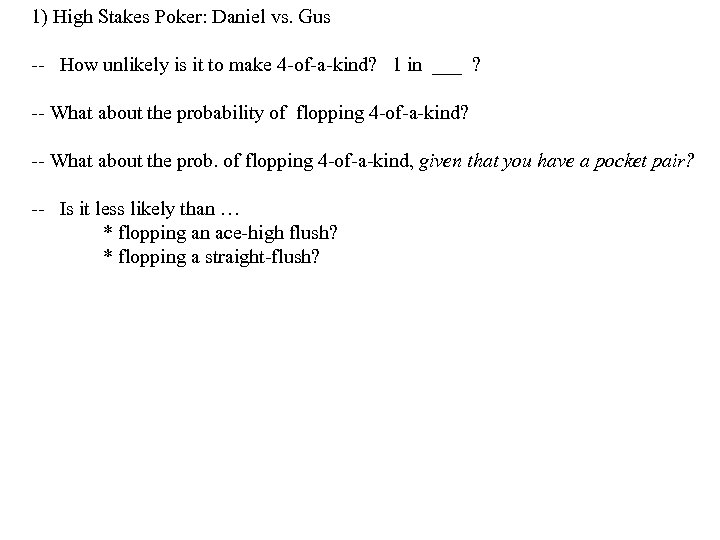1) High Stakes Poker: Daniel vs. Gus -- How unlikely is it to make 4 -of-a-kind? 1 in ___ ? -- What about the probability of flopping 4 -of-a-kind? -- What about the prob. of flopping 4 -of-a-kind, given that you have a pocket pair? -- Is it less likely than … * flopping an ace-high flush? * flopping a straight-flush?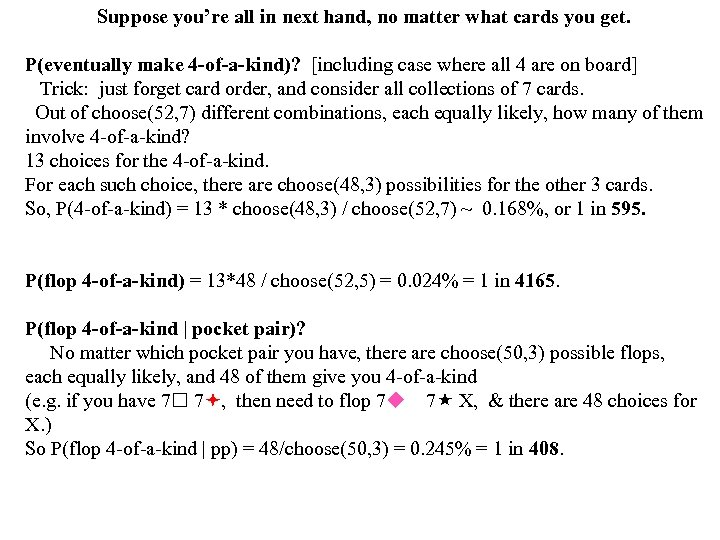Suppose you’re all in next hand, no matter what cards you get. P(eventually make 4 -of-a-kind)? [including case where all 4 are on board] Trick: just forget card order, and consider all collections of 7 cards. Out of choose(52, 7) different combinations, each equally likely, how many of them involve 4 -of-a-kind? 13 choices for the 4 -of-a-kind. For each such choice, there are choose(48, 3) possibilities for the other 3 cards. So, P(4 -of-a-kind) = 13 * choose(48, 3) / choose(52, 7) ~ 0. 168%, or 1 in 595. P(flop 4 -of-a-kind) = 13*48 / choose(52, 5) = 0. 024% = 1 in 4165. P(flop 4 -of-a-kind | pocket pair)? No matter which pocket pair you have, there are choose(50, 3) possible flops, each equally likely, and 48 of them give you 4 -of-a-kind (e. g. if you have 7 7 , then need to flop 7 u 7 X, & there are 48 choices for X. ) So P(flop 4 -of-a-kind | pp) = 48/choose(50, 3) = 0. 245% = 1 in 408.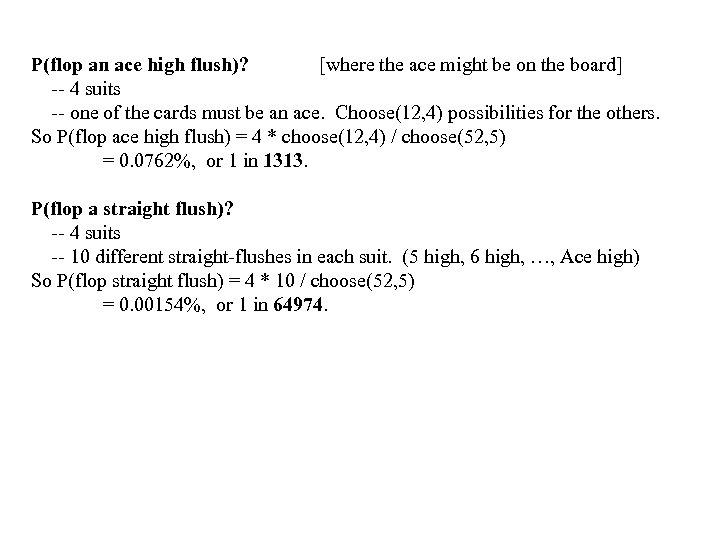P(flop an ace high flush)? [where the ace might be on the board] -- 4 suits -- one of the cards must be an ace. Choose(12, 4) possibilities for the others. So P(flop ace high flush) = 4 * choose(12, 4) / choose(52, 5) = 0. 0762%, or 1 in 1313. P(flop a straight flush)? -- 4 suits -- 10 different straight-flushes in each suit. (5 high, 6 high, …, Ace high) So P(flop straight flush) = 4 * 10 / choose(52, 5) = 0. 00154%, or 1 in 64974.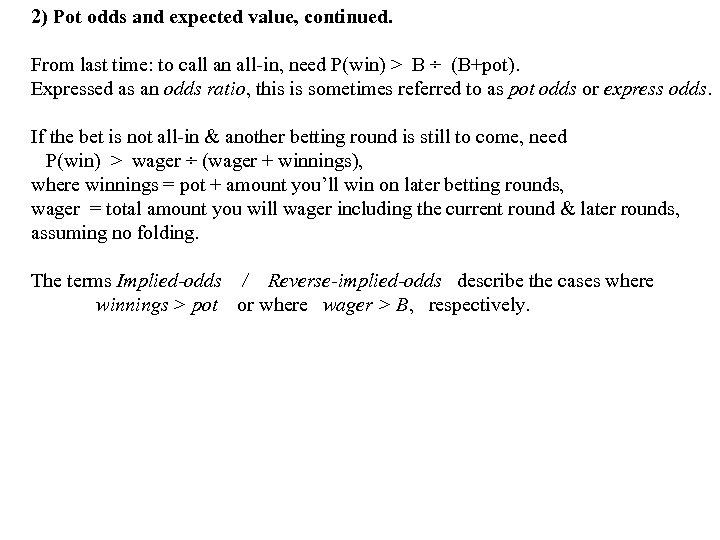2) Pot odds and expected value, continued. From last time: to call an all-in, need P(win) > B ÷ (B+pot). Expressed as an odds ratio, this is sometimes referred to as pot odds or express odds. If the bet is not all-in & another betting round is still to come, need P(win) > wager ÷ (wager + winnings), where winnings = pot + amount you’ll win on later betting rounds, wager = total amount you will wager including the current round & later rounds, assuming no folding. The terms Implied-odds / Reverse-implied-odds describe the cases where winnings > pot or where wager > B, respectively.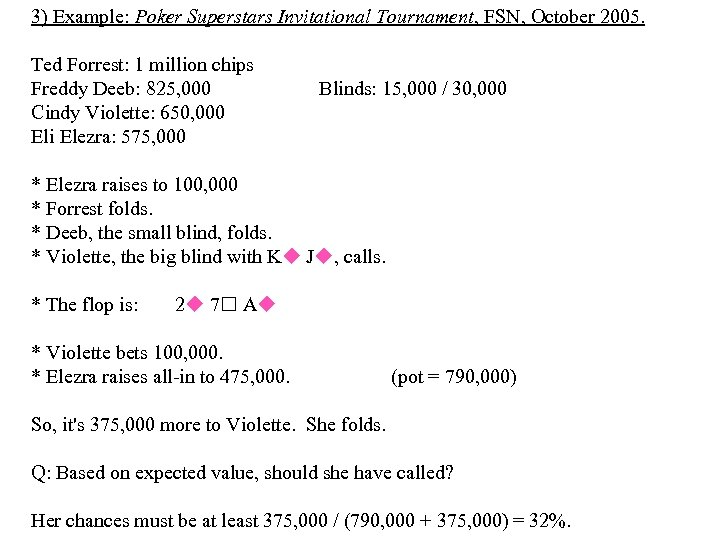3) Example: Poker Superstars Invitational Tournament, FSN, October 2005. Ted Forrest: 1 million chips Freddy Deeb: 825, 000 Cindy Violette: 650, 000 Eli Elezra: 575, 000 Blinds: 15, 000 / 30, 000 * Elezra raises to 100, 000 * Forrest folds. * Deeb, the small blind, folds. * Violette, the big blind with Ku Ju, calls. * The flop is: 2 u 7 Au * Violette bets 100, 000. * Elezra raises all-in to 475, 000. (pot = 790, 000) So, it's 375, 000 more to Violette. She folds. Q: Based on expected value, should she have called? Her chances must be at least 375, 000 / (790, 000 + 375, 000) = 32%. 3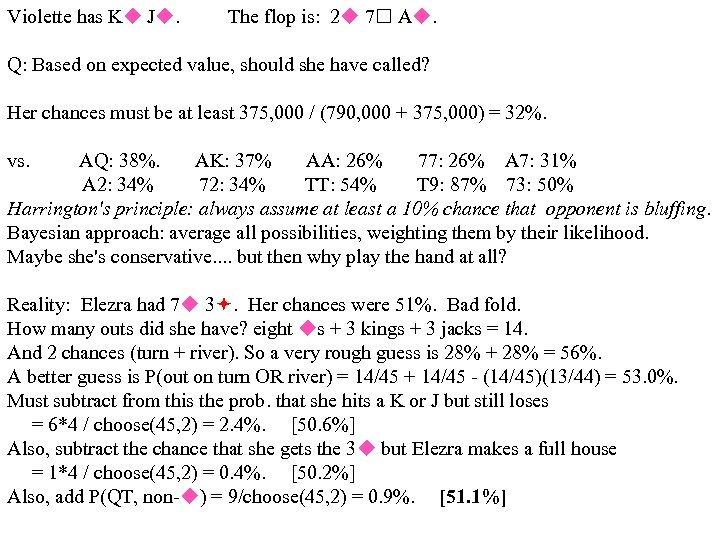Violette has Ku Ju. The flop is: 2 u 7 Au. Q: Based on expected value, should she have called? Her chances must be at least 375, 000 / (790, 000 + 375, 000) = 32%. vs. AQ: 38%. AK: 37% AA: 26% 77: 26% A 7: 31% A 2: 34% 72: 34% TT: 54% T 9: 87% 73: 50% Harrington's principle: always assume at least a 10% chance that opponent is bluffing. Bayesian approach: average all possibilities, weighting them by their likelihood. Maybe she's conservative. . but then why play the hand at all? Reality: Elezra had 7 u 3. Her chances were 51%. Bad fold. How many outs did she have? eight us + 3 kings + 3 jacks = 14. And 2 chances (turn + river). So a very rough guess is 28% + 28% = 56%. A better guess is P(out on turn OR river) = 14/45 + 14/45 - (14/45)(13/44) = 53. 0%. Must subtract from this the prob. that she hits a K or J but still loses = 6*4 / choose(45, 2) = 2. 4%. [50. 6%] Also, subtract the chance that she gets the 3 u but Elezra makes a full house = 1*4 / choose(45, 2) = 0. 4%. [50. 2%] Also, add P(QT, non-u) = 9/choose(45, 2) = 0. 9%. [51. 1%]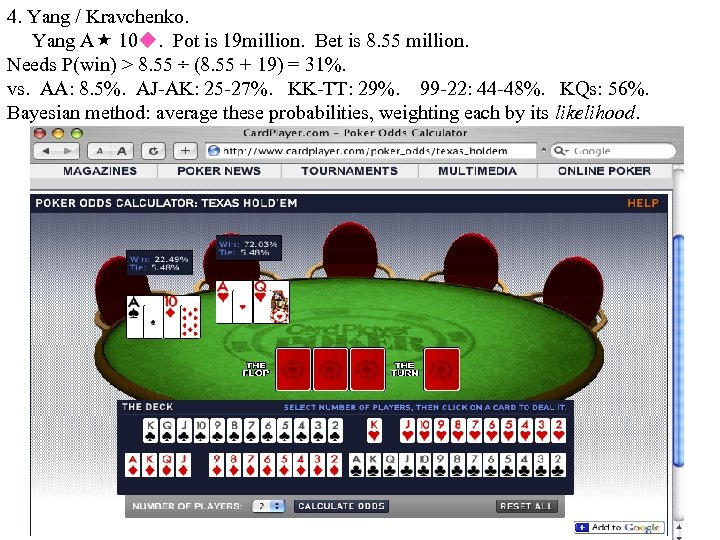4. Yang / Kravchenko. Yang A 10 u. Pot is 19 million. Bet is 8. 55 million. Needs P(win) > 8. 55 ÷ (8. 55 + 19) = 31%. vs. AA: 8. 5%. AJ-AK: 25 -27%. KK-TT: 29%. 99 -22: 44 -48%. KQs: 56%. Bayesian method: average these probabilities, weighting each by its likelihood.